Functions and Algebra: Sketch and interpret functions and graphs

Dylan Busa### Unit outcomes

By the end of this unit you will be able to:

• Identify the following characteristics of quadratic functions:
• turning points
• minima and maxima
• shape and symmetry.
• Sketch and find the equation of the graph $\scriptsize y=ax^2+q$.
• Investigate and generalise the impact of $\scriptsize a$ and $\scriptsize q$ on $\scriptsize y=ax^2+q$.

## What you should know

Before you start this unit, make sure you can

Here is a short self-assessment to make sure you have the skills you need to proceed with this unit.

Solve for $\scriptsize x$ in each case.

1. $\scriptsize 0=3x^2-6$
2. $\scriptsize 2x^2-8=6$

Solutions

1. .
\scriptsize \begin{align*} 0&=3x^2-6\\ \therefore x^2-2& =0\\ \therefore x^2&=2\\ \therefore x&=\pm\sqrt{2} \end{align*}
2. .
\scriptsize \begin{align*} 2x^2-8& =6\\ \therefore 2x^2& =14\\ \therefore x^2&=7\\ \therefore x&=\pm\sqrt{7} \end{align*}

## Introduction

In Unit 1 we learnt that functions are a kind of mathematical relationship between two variables that map each element of the domain (the set of input values) to one, and only one, element in the range (the set of output values).

We learnt that linear functions produce straight lines when we draw their graphs and that the standard form of linear functions is $\scriptsize y=mx+c$ or $\scriptsize y=ax+q$ where:

• $\scriptsize m$ (or $\scriptsize a$) is the gradient or slope of the line, and
• $\scriptsize c$ (or $\scriptsize q$) is the point where the line cuts the y-axis.

So the function $\scriptsize y=3x-1$ or $\scriptsize f(x)=3x-1$, if we write it in function notation, produces a straight line with a gradient of $\scriptsize 3$ that cuts the y-axis at the point $\scriptsize (0,-1)$.

But what kind of graph will the equation $\scriptsize g(x)=4x^2-1$ produce? Will it also be a straight line? Is it even a function? What is the difference between the equations of $\scriptsize f(x)$ and $\scriptsize g(x)$?

In $\scriptsize f(x)$, the highest exponent on $\scriptsize x$ is $\scriptsize 1$. However, in $\scriptsize g(x)$, the highest exponent on $\scriptsize x$ is $\scriptsize 2$. You may, therefore, recognise that $\scriptsize y=4x^2-1$ is a quadratic expression and so $\scriptsize g(x)=4x^2-1$ is a quadratic function.

Of all the functions we will look at, quadratic functions are probably the most important. They produce graphs that we call parabolas. Parabolas crop up everywhere. If you cut a satellite dish in half through the centre, you get a parabola. The same is true for nearly all mirrors used in telescopes, headlamps and some light bulbs.

The ropes or chains hanging from the pillars of suspension bridges follow a parabolic curve.

When you throw a ball (or any object) through the air, its path is a parabola.

All of these examples show us the general shape of the parabola. We can see that parabolas are the graphs of functions. At no point does an input value give more than one output value.There are two important characteristics of parabolas. The first is that they have a turning point; a point where they turn or change direction. The second is that they are symmetrical about the vertical line that goes through their turning point. This means that, if you folded a parabola along this vertical line, the two arms would be exactly on top of each other. We call this line the axis of symmetry.

The turning point of a parabola can either be its highest point or maximum value (like the top of a hill) or its lowest point or minimum value (like the bottom of a valley). When the turning point is the highest point, we call it a maximum turning point. When the turning point is the lowest point, we call it a minimum turning point.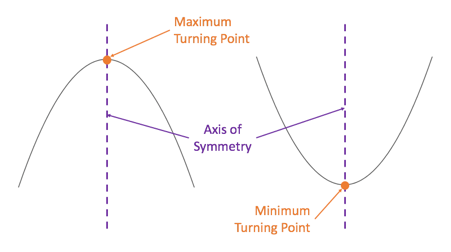### Note

If you have access to the internet, watch the video called “Visual introduction to parabolas” for a good introduction to parabolas.

Visual introduction to parabolas (Duration: 08.15)In this video the turning point is called the vertex. Turning point and Vertex mean the same thing when we are talking about parabolas.

We will now take a closer look at quadratic functions.### Activity 2.1: Investigate the quadratic function

Time required: 30 minutes

What you need:

• a pen or pencil
• a calculator
• blank paper or a notebook

What to do:

Have a look at these two functions:

• $\scriptsize h(x)=2x-3$
• $\scriptsize k(x)=2x^2-3$
1. Which function is the linear function? Why? Which function is the quadratic function? Why?
2. Plot both functions on the same set of axes. You can use any method to plot the linear function. Use the following table of values to help you plot the quadratic function. Plot the points and then join them with as smooth a curve as possible.
 $\scriptsize x$ $\scriptsize -3$ $\scriptsize -2$ $\scriptsize -1$ $\scriptsize 0$ $\scriptsize 1$ $\scriptsize 2$ $\scriptsize 3$ $\scriptsize k(x)$
3. What is the same about each function? What is the same about each graph?
4. What is different about each function? What is different about each graph? Think particularly about the x-intercepts and what you know about the number of answers we get for linear and quadratic equations.
5. If vertical lines have the general form of $\scriptsize y=k$ where $\scriptsize k$ is a constant, what is the equation of the vertical line that is an axis of symmetry of the quadratic function? Remember, the axis of symmetry cuts the graph into two mirror image halves.
6. What is the turning point of the quadratic function?
7. What is the domain and range of the linear function?
8. What is the domain and range of the quadratic function?

What did you find?

1. We were given two similar looking but different functions.
$\scriptsize h(x)$ is the linear function. The highest power on $\scriptsize x$ is $\scriptsize 1$. The function is in the form of a linear expression.
.
$\scriptsize k(x)$ is the quadratic function. The highest power on $\scriptsize x$ is $\scriptsize 2$. The function is in the form of a quadratic expression.
2. Here is the completed table of values for $\scriptsize k(x)$.
 $\scriptsize x$ $\scriptsize -3$ $\scriptsize -2$ $\scriptsize -1$ $\scriptsize 0$ $\scriptsize 1$ $\scriptsize 2$ $\scriptsize 3$ $\scriptsize k(x)$ $\scriptsize 15$ $\scriptsize 5$ $\scriptsize 1$ $\scriptsize 3$ $\scriptsize 1$ $\scriptsize 5$ $\scriptsize 15$

.
Here are the two functions sketched on the same set of axes.
.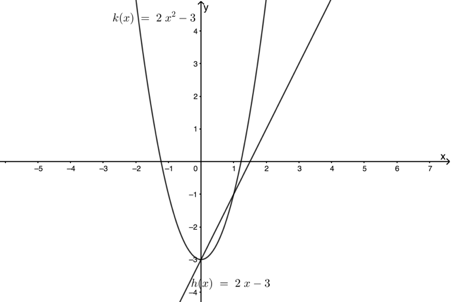3. Each function has a constant term of $\scriptsize -3$ and each graph has a y-intercept of $\scriptsize -3$. Each graph also has a coefficient on the leading term of $\scriptsize 2$.
4. $\scriptsize h(x)$ is a linear function with a highest power of $\scriptsize 1$. It has one intercept with the x-axis (when $\scriptsize y=0$) corresponding to the fact that linear equations only have one root or answer. However, $\scriptsize k(x)$ is a quadratic function with a highest power of $\scriptsize 2$. It has two intercepts with the x-axis (when $\scriptsize y=0$) corresponding to the fact that quadratic equations can have two roots or answers.
5. The parabola is symmetrical about the y-axis. All the $\scriptsize x$ values on the y-axis are zero. Therefore, the axis of symmetry is the line ( $\scriptsize x=0$).
6. The turning point (or TP) of the quadratic function is the point $\scriptsize (0,-3)$.
7. The domain and range of the linear function are both unrestricted. They are both all real numbers.
Domain: $\scriptsize \{x\ |\ x\in \mathbb{R}\}$
Range: $\scriptsize \{h(x)\ |\ h(x)\in \mathbb{R}\}$
8. The domain of the quadratic function is all real numbers. There are no input values (values of $\scriptsize x$) that we cannot use.

The range, however, is different. We can see from the graph that the $\scriptsize y$ values are never less than $\scriptsize -3$. This is the minimum value of the graph. At this point the graph turns around.

So, we can write the domain and range as follows:

Domain: $\scriptsize \{x\ |\ x\in \mathbb{R}\}$
Range: $\scriptsize \{k(x)\ |\ k(x)\in \mathbb{R},k(x)\geq-3\}$

In Activity 2.1 we saw that the value of $\scriptsize q$ in $\scriptsize y=ax+q$ and the quadratic function $\scriptsize y=ax^2+q$ do the same thing – they tell us where the graph cuts the y-axis. This makes sense. Remember that one of the ways to sketch a linear function is the dual-intercept method where we find where the graph intercepts both axes.

• x-intercept (let $\scriptsize y=0$)
• y-intercept (let $\scriptsize x=0$)

Well, the same principle applies to the quadratic function. We can find the y-intercept by letting $\scriptsize x=0$ and we can find the x-intercept by letting $\scriptsize y=0$. Have a look at this example:### Example 2.1

Suppose we have $\scriptsize y=3x^2-1$. Let’s find the intercepts with the axes.

• y-intercept (let $\scriptsize x=0$): $\scriptsize y=3(0)^2-1=-1$. As expected, the y-intercept is simply the value of $\scriptsize q$.
• x-intercept (let $\scriptsize y=0$):

\scriptsize \begin{align*} 0&=3x^2-1\\ \therefore 3x^2&=1\\ \therefore x^2&=\displaystyle \frac{1}{3}\\ \therefore x&=\pm\sqrt\frac{1}{3}} \end{align*

As expected, we have two points where the graph intercepts the x-axis.

The following activity further explores quadratic functions.### Activity 2.2: The effect of a in y=ax2+q

Time required: 30 minutes

What you need:

• a pen or pencil
• a calculator
• blank paper or a notebook
• an internet connection (optional)

What to do:

1. Work through these questions:
1. On the same set of axes, plot the functions $\scriptsize f(x)=2x^2-2$ and $\scriptsize g(x)=\displaystyle \frac{1}{2}x^2-2$. Use a table of values.
2. How does the value of $\scriptsize a$ in $\scriptsize y=ax^2+q$ change the shape of the parabola?
3. What are the turning points of each function? Are these maximum or minimum points?
4. What is the range of each function?
5. Now, on the same set of axes, plot the function $\scriptsize h(x)=-2x^2+2$ and $\scriptsize j(x)=-\displaystyle \frac{1}{2}x^2+2$. Use a table of values.
6. How does changing the sign of $\scriptsize a$ in $\scriptsize y=ax^2+q$ change the shape of the parabola?
7. What are the turning points of each function? Are these maximum or minimum points?
8. What is the range of each function?Here you will find a quadratic function of the form $\scriptsize y=ax^2+q$ with sliders to change the values of $\scriptsize a$ and $\scriptsize q$.
1. Change the value of $\scriptsize q$ to confirm what you know about how the value of $\scriptsize q$ affects the parabola. How does this relate to how $\scriptsize q$ affects the straight line $\scriptsize y=ax+q$?
2. Change the value of $\scriptsize a$ to confirm what you know about how the value of $\scriptsize a$ affects the shape of the parabola. How does this relate to how $\scriptsize a$ affects the straight line $\scriptsize y=ax+q$?
3. What is the difference between a large value of $\scriptsize a$ like $\scriptsize 5$ and a small value of $\scriptsize a$ like $\scriptsize \displaystyle \frac{1}{5}$ on the shape of the parabola?
4. What is the difference between positive and negative values of $\scriptsize a$ on the shape of the parabola?
5. What does a value of $\scriptsize a=0$ mean? Why is this?

What did you find?

1. .
1. Here are functions $\scriptsize f(x)=2x^2-2$ and $\scriptsize g(x)=\displaystyle \frac{1}{2}x^2-2$ plotted on the same set of axes.
.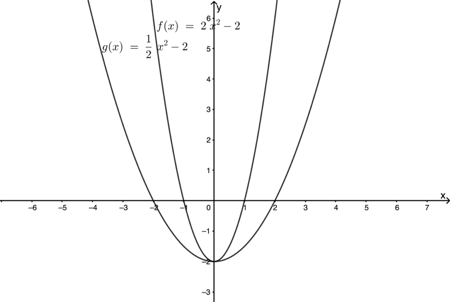2. We can see from the graphs that the bigger the value of $\scriptsize a$, the ‘narrower the parabola. This is the same effect that making the value of $\scriptsize a$ bigger has on the straight line graph given by $\scriptsize y=ax+q$. The greater the value of $\scriptsize a$, the steeper the gradient of the straight line.
3. For each function the turning point is the point $\scriptsize (0,-2)$ (which just so happens to be the y-intercept as well). In both cases, the turning point is a minimum value.
4. The range of each function is all real values greater than or equal to $\scriptsize -2$. We can write the ranges as $\scriptsize \{f(x)\ |\ f(x)\in\mathbb{R},f(x)\geq-2\}$ and $\scriptsize \{g(x)\ |\ g(x)\in\mathbb{R},g(x)\geq-2\}$.
5. Here are the functions $\scriptsize h(x)=-2x^2+2$ and $\scriptsize j(x)=-\displaystyle \frac{1}{2}x^2+2$ plotted on the same set of axes.
.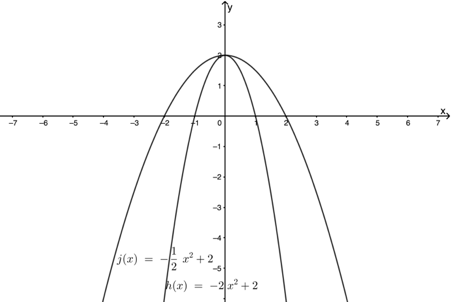6. We can see from the graphs that when the sign of $\scriptsize a$ is changed from positive to negative, the graphs ‘flip over’. Instead of curving upwards they curve downwards. The of $\scriptsize a$ (the value of $\scriptsize a$ irrespective of the sign) still determines the steepness of the graph. The bigger $\scriptsize a$ is, the steeper the graph is.
7. For each function the turning point is the point $\scriptsize (0,2)$ (which happens to be the y-intercept as well). In both cases, the turning point is a maximum value.
8. The range of each function is all real values less than or equal to $\scriptsize 2$. We can write the ranges as $\scriptsize \{f(x)\ |\ f(x)\in\mathbb{R},f(x)\leq2\}$ and $\scriptsize \{g(x)\ |\ g(x)\in\mathbb{R},g(x)\leq2\}$.
2. .
1. In both the quadratic and linear function, the value of $\scriptsize q$ tells us where the graph cuts the y-axis. It gives us the y-intercept.
2. In both the quadratic and linear function, the value of $\scriptsize a$ determines how steep the graph is. A big value of $\scriptsize a$ gives a steep straight line and a steep and narrow parabola.
3. A large value of $\scriptsize a$ gives a very steep and narrow parabola, while a small value of $\scriptsize a$ gives a parabola which is less steep and wider.
4. A positive value of $\scriptsize a$ means that the parabola has a minimum TP and is a ‘smiley face’. A negative value of $\scriptsize a$ gives a parabola which is a ‘sad face’ and has a maximum turning point.
5. When $\scriptsize a = 0$, the quadratic function $\scriptsize y = ax+q$ becomes $\scriptsize y=q$. This is a horizontal straight line where every point on the line has a y-coordinate of $\scriptsize q$.

We learnt from Activity 2.2 that the value of $\scriptsize a$ in $\scriptsize y=ax^2+q$ determines the steepness of the parabola. The greater the value of $\scriptsize a$, the more steep the parabola is. We also discovered that the sign of $\scriptsize a$ (whether it is positive or negative) determines whether the graph bends up into a ‘smiley face’ or down into a ‘sad face’. The absolute value of $\scriptsize a$ (its size irrespective of its sign) still determines how steep or shallow the curve is.

Figure 4 shows a summary of what we have learnt so far for $\scriptsize y=ax^2+q$.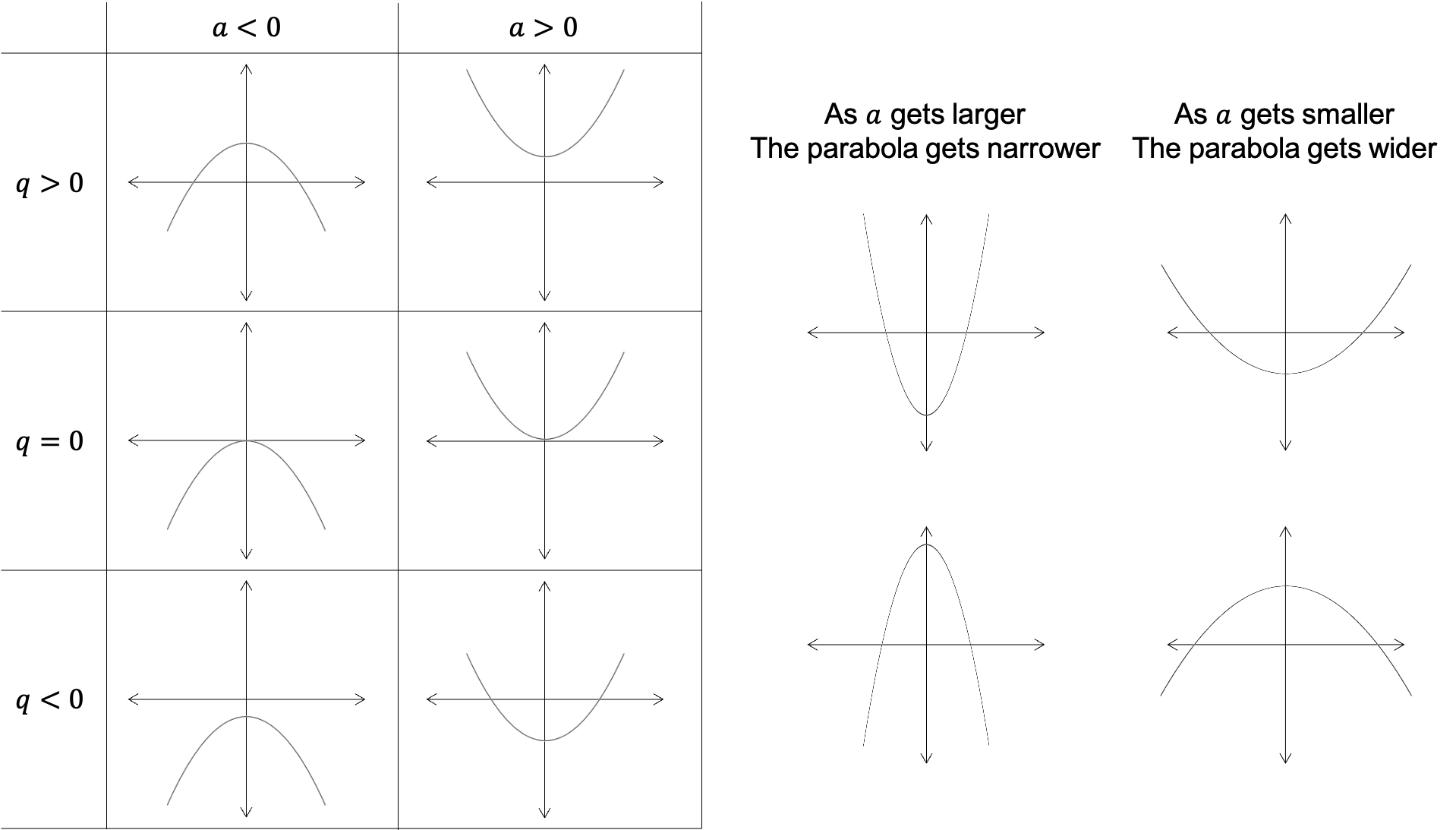Figure 4: Summary of the effects of $\scriptsize a$ and $\scriptsize q$ on the function $\scriptsize y = ax^2=q$.

Look at the summary in Figure 4. Whether the graph has two, one or zero x-intercepts tells us some very important things about the roots of the equation. Remember that ‘roots’ is just another word for the answers to an equation.

In general, we know that quadratic equations have two roots and, therefore, quadratic functions have two x-intercepts. But we can see, for example, when the value of $\scriptsize q=0$ that the TP is on the x-axis and, rather than cutting through the x-axis, the graph just touches the x-axis. In this case, we say the function has one root.

However, when $\scriptsize a\gt0$ and $\scriptsize q\gt0$, we can see that the graph does not even touch the x-axis and there are definitely no x-intercepts. In this case, the quadratic equation has no real roots. The same is true when $\scriptsize a\lt0$ and $\scriptsize q\lt0$.

### Did you know?

When a parabola has no x-intercepts we say that the function has no real roots. The word ‘real’ is important because the function does actually have roots, they are just non-real numbers. The graph will have x-intercepts in the non-real plane. You will learn all about non-real roots in levels three and four.

So the equation $\scriptsize y=3x^2$ (where $\scriptsize q=0$) has one root and the TP of its graph will lie on the x-axis. If we make $\scriptsize y=0$ and solve for $\scriptsize x$ we get:

\scriptsize \begin{align*} 0&=3x^2\\ \therefore 0&=x^2\\ \therefore x&=0 \end{align*}

There is only one root (zero) and the TP, y-intercepts and x-intercept are all on the origin.The equation $\scriptsize y=3x^2+2$ (where $\scriptsize a\gt0$ and $\scriptsize q\gt0$) has no real roots and its graph will have no x-intercepts. If we make $\scriptsize y=0$ and solve for $\scriptsize x$ we get:

\scriptsize \begin{align*} 0&=3x^2+2\\ \therefore 3x^2&=-2\\ \therefore x^2&=-\displaystyle \frac{2}{3} \end{align*}

But there is no number we know of that, when we multiply it by itself, will give us a negative number ($\scriptsize -\displaystyle \frac{2}{3}$ in this case) so there are no real solutions to the equation and the graph will not cut or even touch the x-axis.### Example 2.2

State whether the graphs of the following quadratic equations will have two, one or zero x-intercepts.

1. $\scriptsize y=2x^2-7$
2. $\scriptsize y=-2x^2-7$
3. $\scriptsize -7y=-2x^2-7$
4. $\scriptsize 3y-4x^2=0$

Solution

1. The value of $\scriptsize a$ is positive and the value of $\scriptsize q$ is negative. Therefore, the graph will have $\scriptsize 2$ x-intercepts.
2. The value of $\scriptsize a$ is negative and the value of $\scriptsize q$ is negative. Therefore, the graph will have no x-intercepts.
3. We need to get the equation into standard form:
.
\scriptsize \begin{align*} -7y&=-2x^2-7\\ \therefore y&=\displaystyle \frac{-2}{-7}x^2+1\\ \therefore y&=\displaystyle \frac{2}{7}x^2+1 \end{align*}
.
The value of $\scriptsize a$ is positive and the value of $\scriptsize q$ is positive. Therefore, the graph will have no x-intercepts.
4. We need to get the equation into standard form.
.
\scriptsize \begin{align*} 3y-4x^2& =0\\ \therefore 3y&=4x^2\\ \therefore y&=\displaystyle \frac{4}{3}x^2\\ \end{align*}
.
The value of $\scriptsize a$ is positive and $\scriptsize q=0$. Therefore, the graph will have $\scriptsize 1$ x-intercept. In other words, it will touch the x-axis.

Now, have a look at the next example to get some practise sketching quadratic functions of the form $\scriptsize y=ax^2+q$.### Example 2.3

1. Sketch the function $\scriptsize f(x)=3x^2-12$. Mark the TP and intercepts.
1. Is the TP a maximum or minimum?
2. State the domain and range of the function.
3. What is the axis of symmetry?
2. Sketch the function $\scriptsize h(x)=-\displaystyle \frac{1}{2}x^2-3$. Mark the intercepts and TP.
1. Is the TP a maximum of minimum?
2. State the domain and range of the function.
3. What is the axis of symmetry?
3. Given $\scriptsize s(x)=-3x^2+7$ and $\scriptsize t(x)=\displaystyle \frac{1}{3}x^2+3$. Without sketching the functions, determine:
1. Which graph will be steeper?
2. Which function has no real roots?
3. Which function has a minimum TP? What is the TP?

Solution

1. Here is the sketch of $\scriptsize f(x)=3x^2-12$ with the TP and intercepts marked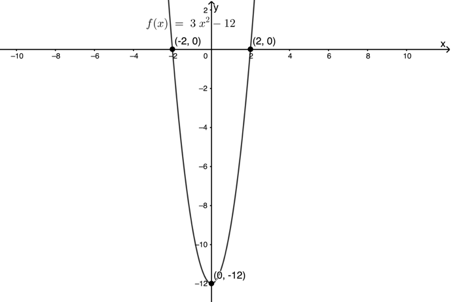1. The TP is a minimum.
2. Domain: $\scriptsize \{x\ |\ x\in\mathbb{R}\}$
Range: $\scriptsize \{f(x)\ |\ f(x)\in\mathbb{R},f(x)\geq-12\}$
3. The axis of symmetry is the y-axis or the line $\scriptsize x=0$.
2. Here is the sketch of $\scriptsize h(x)=-\displaystyle \frac{1}{2}x^2-3$. Note that because the graph has no x-intercepts, we needed to find other points to help us accurately draw the shape of the graph.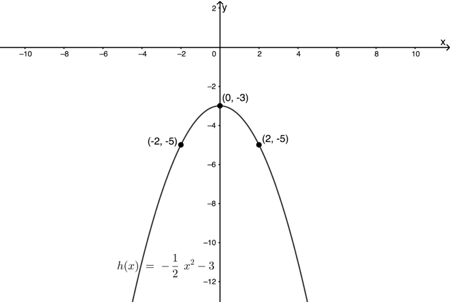1. The TP is a maximum.
2. Domain: $\scriptsize \{x\ |\ x\in\mathbb{R}\}$
Range: $\scriptsize \{h(x)\ |\ h(x)\in\mathbb{R},h(x)\leq-3\}$
3. The axis of symmetry is the y-axis or the line $\scriptsize x=0$.
3. The functions are $\scriptsize s(x)=-3x^2+7$ and $\scriptsize t(x)=\displaystyle \frac{1}{3}x^2+3$
1. The absolute value of $\scriptsize a$ in $\scriptsize s(x)$ is $\scriptsize 3$ while in $\scriptsize t(x)$ it is $\scriptsize \displaystyle \frac{1}{3}$. Therefore $\scriptsize s(x)$ will be the steeper curve.
2. $\scriptsize t(x)$ will have no real roots because the value of $\scriptsize a$ is positive and the value of $\scriptsize q$ is positive.
3. In $\scriptsize t(x)$, the value of $\scriptsize a$ is positive, therefore the graph curves upwards and has a minimum TP. Because the function is of the form $\scriptsize y=ax^2+q$, the TP will be the same as the y-intercept which is $\scriptsize 3$.### Exercise 2.1

1. Look at the Cartesian plane below and match each of the equations to one of the graphed functions.
1. $\scriptsize y=-2x^2$
2. $\scriptsize y=3x^2+1$
3. $\scriptsize y=\displaystyle \frac{1}{2}x^2$
4. $\scriptsize y=x^2$
5. $\scriptsize y=-4x^2$
6. $\scriptsize y=3x^2-2$
.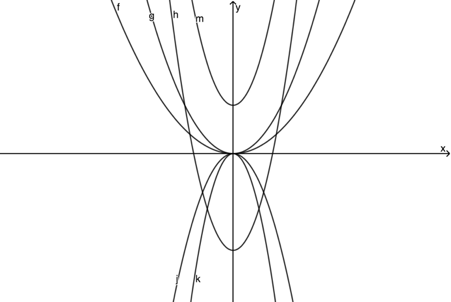2. Sketch the function $\scriptsize q(x)=-\displaystyle \frac{2}{3}x^2+6$. Mark the TP and intercepts.
1. State the domain and range of $\scriptsize q(x)$.
2. What is the equation of the axis of symmetry?
3. Without sketching the graph, determine what the TP of $\scriptsize 4y+3x^2=-48$ is and whether it is a maximum or minimum TP.
4. Sketch the function given by $\scriptsize c(x)=3x^2-4$ as accurately as possible showing the intercepts, TP and axis of symmetry on your sketch.

The full solutions are at the end of the unit.

Let’s quickly summarise what we know about quadratic functions of the form $\scriptsize y=ax^2+q$ and how to sketch them.

In order to sketch graphs of the form $\scriptsize y=ax^2+q$, we need to determine the following:

• The sign of $\scriptsize a$: this tells us if the graph curves upwards and has a minimum TP or whether it curves downwards and has a maximum TP.
• The y-intercept: we find this by letting $\scriptsize x=0$.
• The x-intercepts: we find these by letting $\scriptsize y=0$ (Note: there may be no x-intercepts. In this case, it is necessary to find one or two other points on the graph to help us get the shape right.)
• The TP: for $\scriptsize y=ax^2+q$ this is always the same as the y-intercept.

# Find the equation of quadratic functions

Now that we can sketch quadratic functions, we need to see how to find their equations from their graphs.### Example 2.4

1. The image below shows a graph of a function of the form $\scriptsize y=ax^2+q$. Point $\scriptsize { \text{A}}$ is the turning point with coordinates $\scriptsize (0,4)$ and point $\scriptsize { \text{B}}$ is $\scriptsize (-2,\displaystyle \frac{8}{3})$.
..
Find the equation of the graph.
2. Find the equation of the function passing through the point $\scriptsize (-3,-3\displaystyle \frac{3}{5})$ with a TP on the origin and an axis of symmetry of $\scriptsize x=0$.

Solution

1. We are told that the function is of the form $\scriptsize y=ax^2+q$. Therefore, we know that the TP and the y-intercept are the same point. We are told that the TP is the point $\scriptsize \text{A}(0,4)$.
Therefore $\scriptsize q=4$.
.
So far, we can write the function as $\scriptsize y=ax^2+4$. Now substitute the coordinates of $\scriptsize \text{B}$ into the equation and solve for $\scriptsize a$.
.
\scriptsize \begin{align*} \displaystyle \frac{8}{3}&=a(-2)^2+4\\ \therefore 8&=12a+12\\ \therefore 12a&=-4\\ \therefore a&=-\displaystyle \frac{1}{3} \end{align*}
.
So the equation of the graph is $\scriptsize y=-\displaystyle \frac{1}{3}x^2+4$ .
2. We are told that the axis of symmetry is the line $\scriptsize x=0$. Therefore, the function is of the form $\scriptsize y=ax^2+q$. We are also told that the TP is on the origin. This means that the y-intercept is $\scriptsize 0$ and, therefore, $\scriptsize q=0$. So, the equation of the function is $\scriptsize y=ax^2$.
.
We can substitute $\scriptsize (-3,-3\displaystyle \frac{3}{5})$ into this equation for $\scriptsize x$ and $\scriptsize y$ and solve for $\scriptsize a$.
.
\scriptsize \begin{align*} -3\displaystyle \frac{3}{5}&=a(-3)^2\\ \therefore \displaystyle \frac{-18}{5}&=9a\\ \therefore -18&=45a\\ \therefore a&=-\displaystyle \frac{18}{45}\\ \therefore a&=-\displaystyle \frac{2}{5} \end{align*}
.
So the equation of the function is $\scriptsize y=-\displaystyle \frac{2}{5}x^2$ .### Exercise 2.2

1. Find the equation of the graph of the form $\scriptsize y=ax^2+q$ that passes through the origin and the point $\scriptsize (3,-\displaystyle \frac{4}{3})$.
2. The functions $\scriptsize r(x)$ and $\scriptsize q(x)$, both of the form $\scriptsize y=ax^2+q$, are plotted as shown. They intersect each other at $\scriptsize \text{P}(\displaystyle \frac{3}{\sqrt{2}},5)$. $\scriptsize \text{S}$ is the point $\scriptsize (0,8)$ and $\scriptsize \text{T}$ is the point $\scriptsize (0,-4)$.
.1. Find the equations of both functions.
2. For which values of $\scriptsize x$ is $\scriptsize r$ increasing? In other words, for which values of $\scriptsize x$ are the y-values of $\scriptsize r$ getting bigger and bigger?
3. For which values of $\scriptsize x$ is $\scriptsize q(x)\gt r(x)$?
3. The range of $\scriptsize d(x)$ is given as $\scriptsize [-4,\infty]$. If $\scriptsize d(x)$ is a quadratic function of the form $\scriptsize y=ax^2+q$ and passes through the point $\scriptsize (1,3)$, what is the equation of $\scriptsize d(x)$?

The full solutions are at the end of the unit.

## Summary

In this unit you have learnt the following:

• The function of the form $\scriptsize y=ax^2+q$ is a parabola with an axis of symmetry of the y-axis or the line $\scriptsize x=0$ and a y-intercept of $\scriptsize (0,q)$ .
• Because the axis of symmetry is the line $\scriptsize x=0$, the turning point (TP) and the y-intercept are the same point; therefore the TP is the point $\scriptsize (0,q)$.
• If $\scriptsize a\gt0$, the graph curves upwards and has a minimum TP.
• If $\scriptsize a\lt0$, the graph curves downwards and has a maximum TP.
• If $\scriptsize a\lt0$ and $\scriptsize q\lt0$, or $\scriptsize a\gt0$ and $\scriptsize q\gt0$, the graph has no x-intercepts and the equation has no real roots.
• If $\scriptsize q=0$, the graph touches the x-axis and the equation has one real root.

# Unit 2: Assessment

#### Suggested time to complete: 30 minutes

1. Determine whether $\scriptsize 2x^2-3y=4$, has a minimum or maximum value and give the corresponding value of $\scriptsize x$.
2. From the following sketch:
.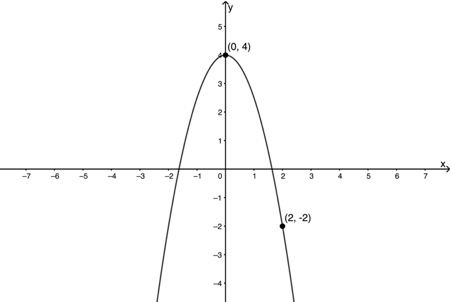1. Determine the range for the parabola $\scriptsize f(x)$.
2. Determine the domain for the parabola $\scriptsize f(x)$.
3. Determine the equation for the parabola $\scriptsize f(x)$.
3. Given $\scriptsize y=2-2x^2$, determine the following:
1. The x-intercepts.
2. The y-intercepts.
3. The turning point.
4. The axis of symmetry
5. The range of the graph.
6. The domain of the graph.
7. Sketch the graph, showing the TP and all intercepts.

The full solutions are at the end of the unit.

# Unit 2: Solutions

### Exercise 2.1

1. .
1. $\scriptsize y=-2x^2$: $\scriptsize j(x)$
2. $\scriptsize y=3x^2+1$: $\scriptsize m(x)$
3. $\scriptsize y=\displaystyle \frac{1}{2}x^2$: $\scriptsize f(x)$
4. $\scriptsize y=x^2$: $\scriptsize g(x)$
5. $\scriptsize y=-4x^2$: $\scriptsize k(x)$
6. $\scriptsize y=3x^2-2$: $\scriptsize h(x)$
2. .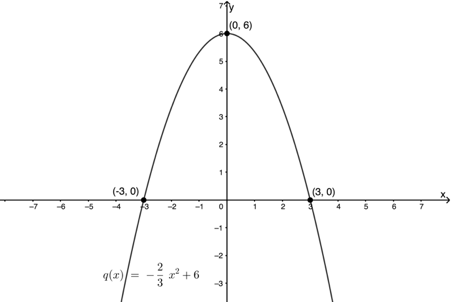1. Domain: $\scriptsize \{x\ |\ x\in\mathbb{R}\}$
Range: $\scriptsize \{q(x)\ |\ q(x)\in\mathbb{R},q(x)\leq6\}$
2. The axis of symmetry is the y-axis or the line $\scriptsize x=0$.
3. .
\scriptsize \begin{align*} 4y+3x^2&=-48\\ \therefore 4y&=-3x^2-48\\ \therefore y&=-\displaystyle \frac{3}{4}x^2-12 \end{align*}
.
Because the function is of the form $\scriptsize y=ax^2+q$ the TP is the same as the y-intercept. Therefore, the TP is the point $\scriptsize (0,-12)$.
.
The value of $\scriptsize a$ is negative, therefore the graph curves downwards and the TP is a maximum.
4. .Back to Exercise 2.1

### Exercise 2.2

1. The graph passes through the origin. Therefore $\scriptsize q=0$.
Substitute $\scriptsize (3,-\displaystyle \frac{4}{3})$ into $\scriptsize y=ax^2$ to solve for $\scriptsize a$.
.
\scriptsize \begin{align*} -\displaystyle \frac{4}{3}&=a(3)^2\\ \therefore-4&=27a\\ \therefore a&=-\displaystyle \frac{4}{27} \end{align*}
.
The function is $\scriptsize y=-\displaystyle \frac{4}{27}x^2$.
2. .
1. $\scriptsize r(x)$:
The y-intercept of the function is $\scriptsize (0,-4)$. Therefore $\scriptsize q=-4$.
Substitute $\scriptsize \text{P}(\displaystyle \frac{3}{\sqrt{2}},5)$ into $\scriptsize y=ax^2-4$.
.
\scriptsize \begin{align*} 5&=a\left(\displaystyle \frac{3}{\sqrt{2}}\right)^2-4\\ \therefore5&=\left(\displaystyle \frac{9}{2}\right)a-4\\ \therefore 10&=9a-8\\ \therefore 18&=9a\\ \therefore a&=2 \end{align*}
$\scriptsize \therefore r(x)=2x^2-4$
.
$\scriptsize q(x)$:
The y-intercept of the function is $\scriptsize (0,8)$. Therefore $\scriptsize q=-8$.
Substitute $\scriptsize \text{P}(\displaystyle \frac{3}{\sqrt{2}},5)$ into $\scriptsize y=ax^2+8$.
\scriptsize \begin{align*} 5&=a\left(\displaystyle \frac{3}{\sqrt{2}}\right)^2+8\\ \therefore5&=\left(\displaystyle \frac{9}{2}\right)a+8\\ \therefore 10&=9a+16\\ \therefore -6&=9a\\ \therefore a&=-\displaystyle \frac{2}{3} \end{align*}
$\scriptsize \therefore q(x)=-\displaystyle \frac{2}{3}x^2+8$
2. $\scriptsize r(x)$ increases for all values to the right of the minimum TP. Therefore, $\scriptsize r(x)$ increases for $\scriptsize x\geq0$.
3. $\scriptsize q(x)\gt r(x)$ between the points where the graphs intersect. Because the graphs have an axis of symmetry of $\scriptsize x=0$, they intersect at the points $\scriptsize (\displaystyle \frac{3}{\sqrt{2}},5)$ and $\scriptsize (-\displaystyle \frac{3}{\sqrt{2}},5)$. Therefore, $\scriptsize q(x)\gt r(x)$ for $\scriptsize \left(-\displaystyle \frac{3}{\sqrt{2}},\displaystyle \frac{3}{\sqrt{2}}\right)$.
.
Note: We need to use round brackets to indicate that the end points of the interval are not included because we are only looking for where $\scriptsize q(x)\gt r(x)$.
3. The range of $\scriptsize d(x)$ is given as $\scriptsize [-4,\infty]$ and the function is of the form $\scriptsize y=ax^2+q$. Therefore, it has a minimum TP and y-intercept of $\scriptsize (-4,0)$ . Therefore $\scriptsize q=-4$.
Substitute $\scriptsize (1,3)$ into $\scriptsize y=ax^2-4$ and solve for $\scriptsize a$.
\scriptsize \begin{align*} 3&=a(1)^2-4\\ \therefore 3&=a-4\\ \therefore a&=7 \end{align*}
.
$\scriptsize \therefore d(x)=7x^2-4$

Back to Exercise 2.2

### Unit 2: Assessment

1. .
\scriptsize \begin{align*} 2x^2-3y&=4\\ \therefore -3y& =-2x^2+4\\ \therefore y& =\displaystyle \frac{2}{3}-\displaystyle \frac{4}{3} \end{align*}
$\scriptsize \therefore a\gt0$ so the TP is a minimum and the corresponding $\scriptsize x$ value of the TP is $\scriptsize x=0$ (the axis of symmetry is the line $\scriptsize x=0$).
2. .
1. Range: $\scriptsize \{f(x)\ |\ f(x)\in\mathbb{R},f(x)\leq4\}$
2. Domain: $\scriptsize \{x\ |\ x\in\mathbb{R}\}$
3. $\scriptsize f(x)=ax^2+4$. Substitute $\scriptsize (2,-2)$ into $\scriptsize f(x)$ to solve for $\scriptsize a$:
\scriptsize \begin{align*} -2&=a(2)^2+4\\ \therefore -2& =4a+4\\ \therefore 4a&=-6\\ \therefore a&=-\displaystyle \frac{3}{2} \end{align*}
$\scriptsize \therefore f(x)=-\displaystyle \frac{3}{2}x^2+4$.
3. .
1. x-intercepts (let $\scriptsize y=0)$:
\scriptsize \begin{align*} 0&=2-2x^2\\ \therefore 2x^2&=2\\ \therefore x^2&=1\\ \therefore x&=\pm1 \end{align*}
Therefore x-intercepts are $\scriptsize (-1,0)$ and $\scriptsize (1,0)$.
2. y-intercepts (let $\scriptsize x=0)$:
\scriptsize \begin{align*} y&=2-2(0)^2\\ \therefore y&=2 \end{align*}
Therefore y-intercept is the point $\scriptsize (0,2)$.
3. TP is the point $\scriptsize (0,2)$.
4. The axis of symmetry is the line $\scriptsize x=0$.
5. $\scriptsize a\lt0$ therefore the TP is a maximum.
Range: $\scriptsize \{y\ |\ y\in\mathbb{R},y\leq2\}$
6. Domain: $\scriptsize \{x\ |\ x\in\mathbb{R}\}$
7. .Back to Unit 2: Assessment